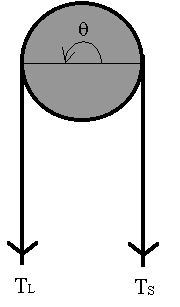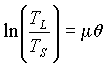# Mechanical: Rope Tension and Logarithms

When a rope is partially wrapped around a fixed drum with a force at each end, as shown in the figure, the relationship between the larger tension TL and the smaller tension Ts is given by(1)

where θ is the angle of wrap in radians and μ is the coefficient of friction. Another form of this equation is(2)

We have to be careful about the meaning of TL and TS when applying formulas (1) and (2) to real situations. The direction of motion, or the tendency of motion, is determined by the larger tension TL . The friction between the rope and the drum is always against the relative motion. In other words, the friction always "helps" the smaller side. For example, if you try to hold a box hanging at the other end of the rope, the force you need is the smaller tension TS as the frictional force can help you. However, if you try to lift the box, the force you need would be the larger tension TL because now the frictional force is against you.

## Questions:

1. In the mechanism schematically shown in the figure, assuming the coefficient of static friction is 0.2, what will be the force needed to hold a 300lb box?
2. What will be the force needed to lift the box?
3. If we wrap the rope around the drum one more turn (now 1.5 turns), what would be the answers for the previous two questions?
4. Derive equation (2) from equation (1).
5. Derive equation (1) from equation (2).Written by Michael Chen, October 7, 1997